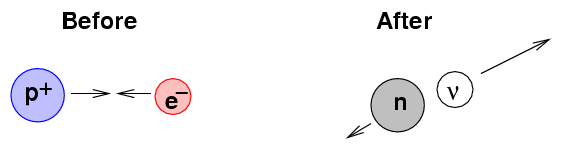# What are some common radioisotopes that decay by electron capture?

Aug 28, 2014

Electron capture is a process in which a nucleus with too many protons absorbs an electron from an inner orbital

This changes a proton to a neutron and simultaneously emits an electron neutrino.

Here are some examples.

""_-1^0"e" + _4^7"Be" → _3^7"Li" + ν_e

""_-1^0"e" + _13^26"Al" → _12^26"Mg" + ν_e

""_-1^0"e" + _19^40"K" → _18^40"Ar" + ν_e

""_-1^0"e" + _28^59"Ni" → _27^59"Co" + ν_e

""_-1^0"e" + _37^83"Rb" → _36^83"Kr" + ν_eIn each case, we lose a proton, but we gain a neutron. The atomic mass stays the same, but the new element has one atomic number less than the original had.